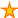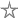# VBA: Convert text to proper case (alternative to StrConv())

10 votes:16,964 views      No comments
by Allen Browne, 20 April 2005    (for Access v1, v2)

### Proper()

Use this function to correct case for proper nouns. For example, if company names have been entered in all upper case, create an Update Query that calls this function to correct it. You will probably need to manually correct those that don't follow the rule: the function does not handle names like McDonald or van Leen

```Function Proper (var As Variant) As Variant
' Purpose: Convert the case of var so that the first letter of each word capitalized.
Dim strV As String, intChar As Integer, i As Integer
Dim fWasSpace As Integer    'Flag: was previous char a space?

If IsNull(var) Then Exit Function
strV = var
fWasSpace = True              'Initialize to capitalize first letter.
For i = 1 To Len(strV)
intChar = Asc(Mid\$(strV, i, 1))
Select Case intChar
Case 65 To 90              ' A to Z
If Not fWasSpace Then Mid\$(strV, i, 1) = Chr\$(intChar Or &H20)
Case 97 To 122             ' a to z
If fWasSpace Then Mid\$(strV, i, 1) = Chr\$(intChar And &HDF)
End Select
fWasSpace = (intChar = 32)
Next
Proper = strV
End Function```

Rate this article: